# 1 4 Trigonometry Sine Cosine and Tangent Sine

• Slides: 21
Download presentation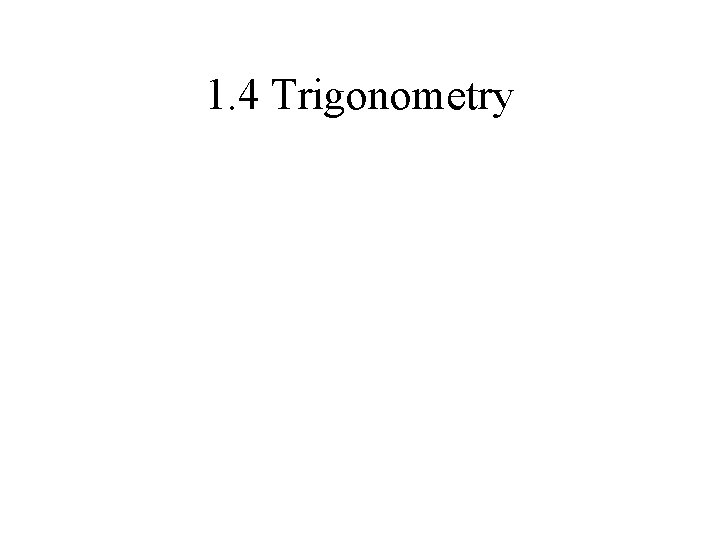1. 4 TrigonometrySine, Cosine, and TangentSine, Cosine, and Tangent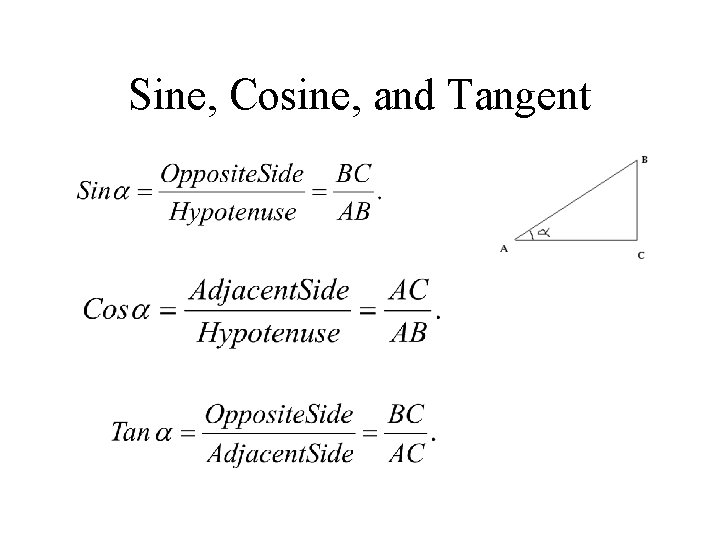Sine, Cosine, and Tangent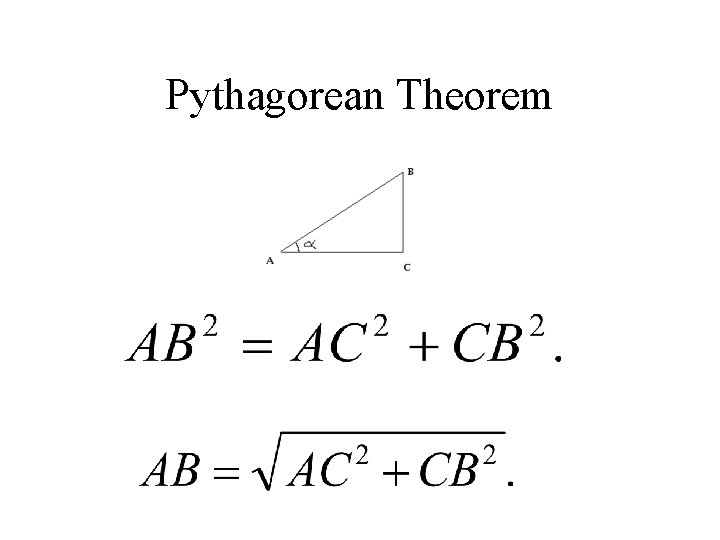Pythagorean Theorem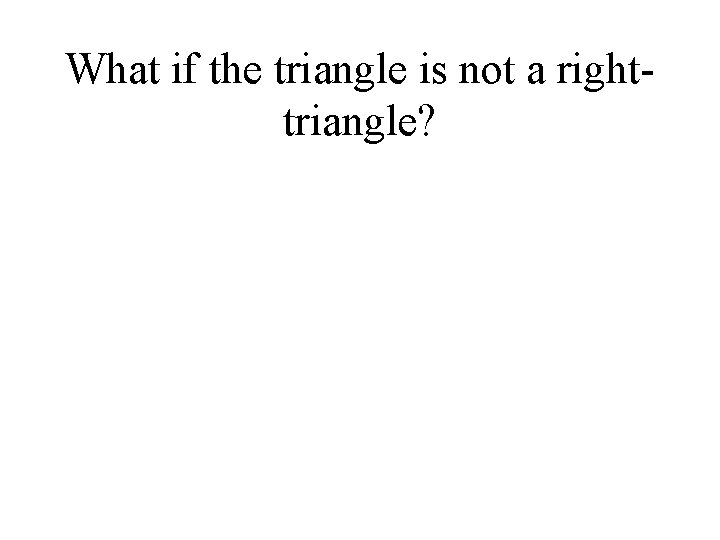What if the triangle is not a righttriangle?Law of Cosines and Law of Sines Law of cosines is given by, Law of sines is given by,1. 5 Scalars and Vectors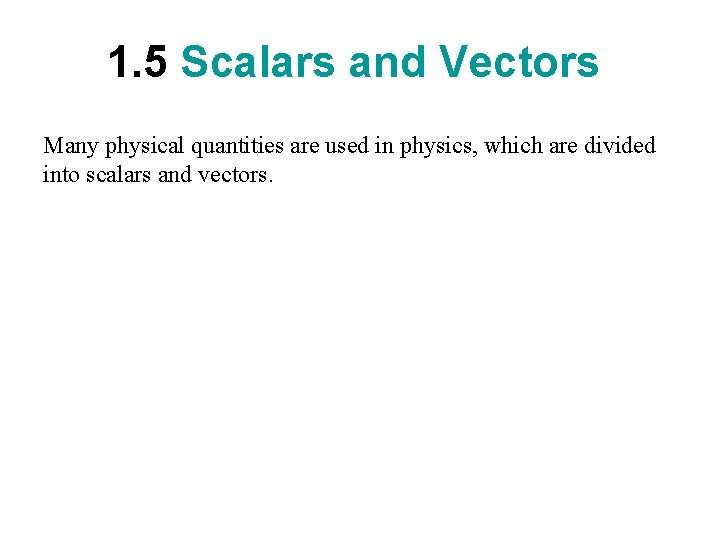1. 5 Scalars and Vectors Many physical quantities are used in physics, which are divided into scalars and vectors.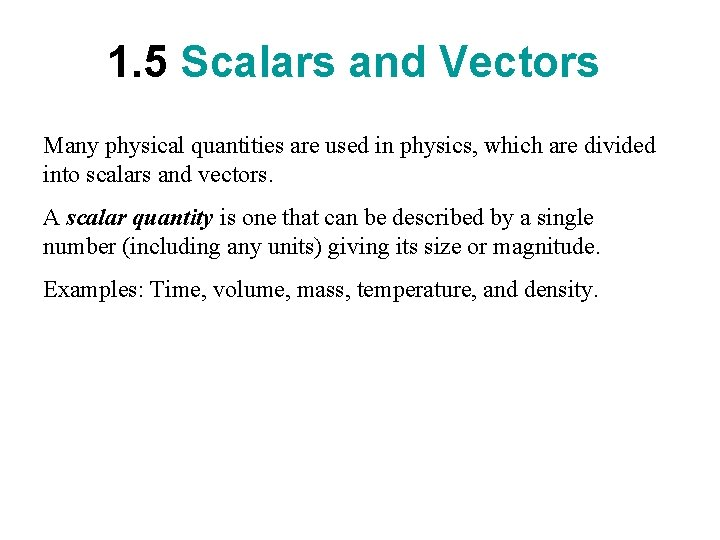1. 5 Scalars and Vectors Many physical quantities are used in physics, which are divided into scalars and vectors. A scalar quantity is one that can be described by a single number (including any units) giving its size or magnitude. Examples: Time, volume, mass, temperature, and density.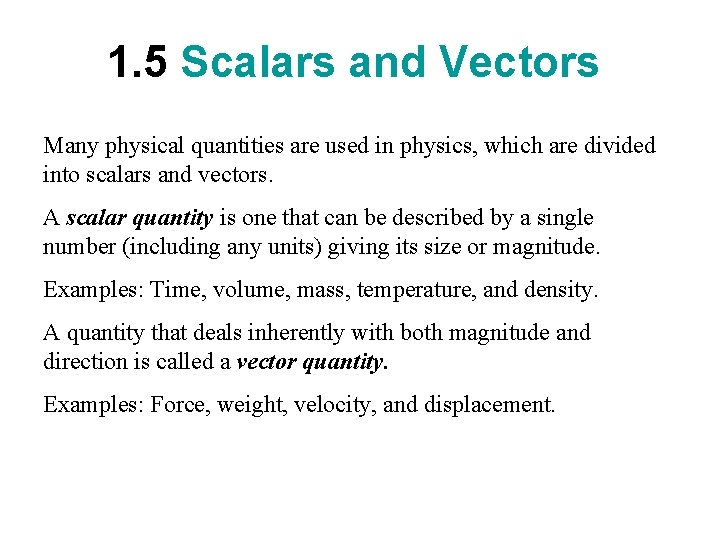1. 5 Scalars and Vectors Many physical quantities are used in physics, which are divided into scalars and vectors. A scalar quantity is one that can be described by a single number (including any units) giving its size or magnitude. Examples: Time, volume, mass, temperature, and density. A quantity that deals inherently with both magnitude and direction is called a vector quantity. Examples: Force, weight, velocity, and displacement.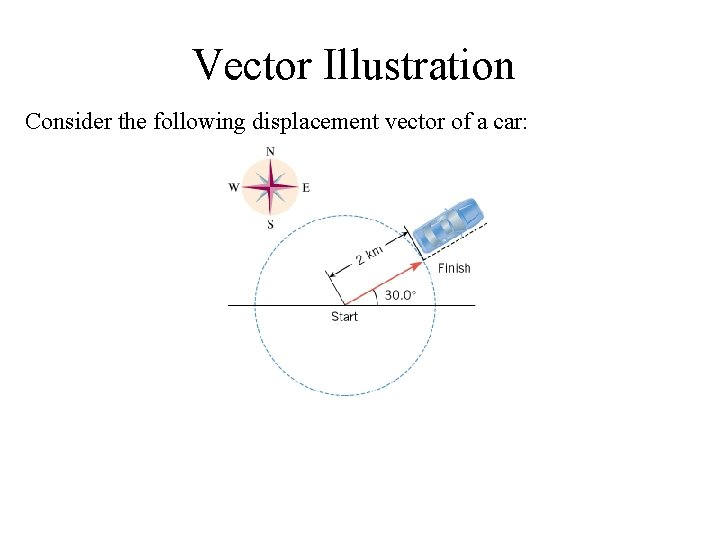Vector Illustration Consider the following displacement vector of a car: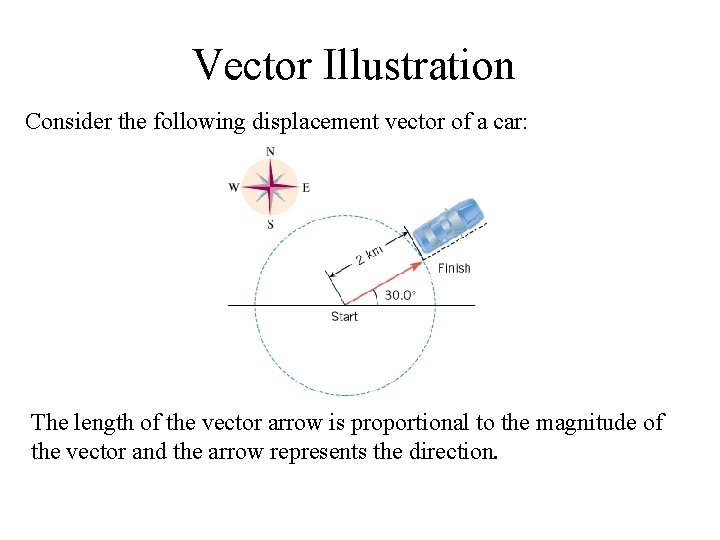Vector Illustration Consider the following displacement vector of a car: The length of the vector arrow is proportional to the magnitude of the vector and the arrow represents the direction.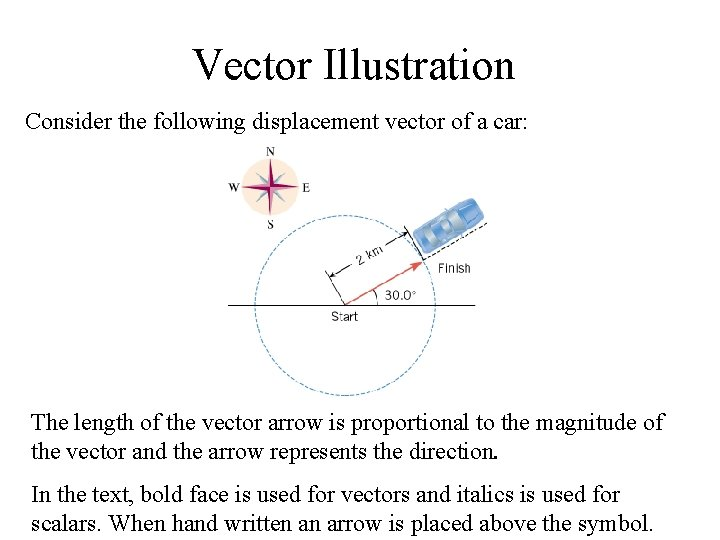Vector Illustration Consider the following displacement vector of a car: The length of the vector arrow is proportional to the magnitude of the vector and the arrow represents the direction. In the text, bold face is used for vectors and italics is used for scalars. When hand written an arrow is placed above the symbol.1. 6 VECTOR ADDITION Addition of two co-linear displacement vectors A and B, both are due East. (A = 275 -m, B = 125 -m)1. 6 VECTOR ADDITION What if vector B (125 -m) is due West?1. 6 VECTOR ADDITION What if vector B is 275 -m, due West?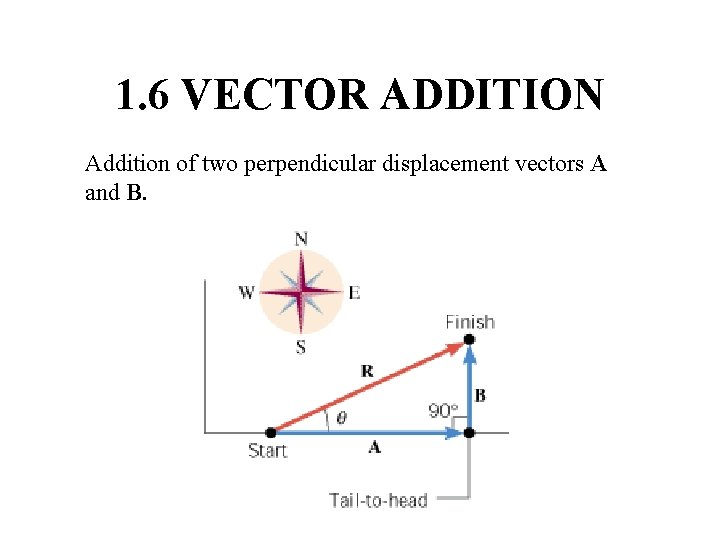1. 6 VECTOR ADDITION Addition of two perpendicular displacement vectors A and B.1. 6 VECTOR ADDITION Addition of two displacement vectors A and B, neither colinear nor perpendicular, using graphical method.1. 6 VECTOR ADDITION Addition of two displacement vectors A and B, neither colinear nor perpendicular, using laws of sines and cosines.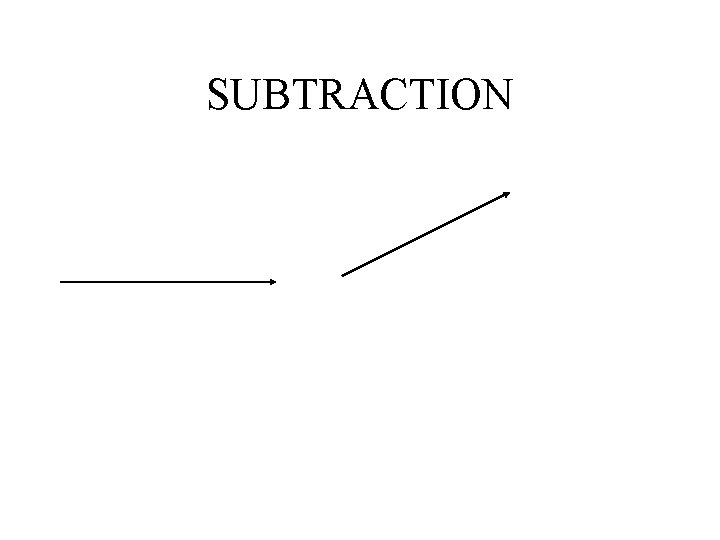SUBTRACTION